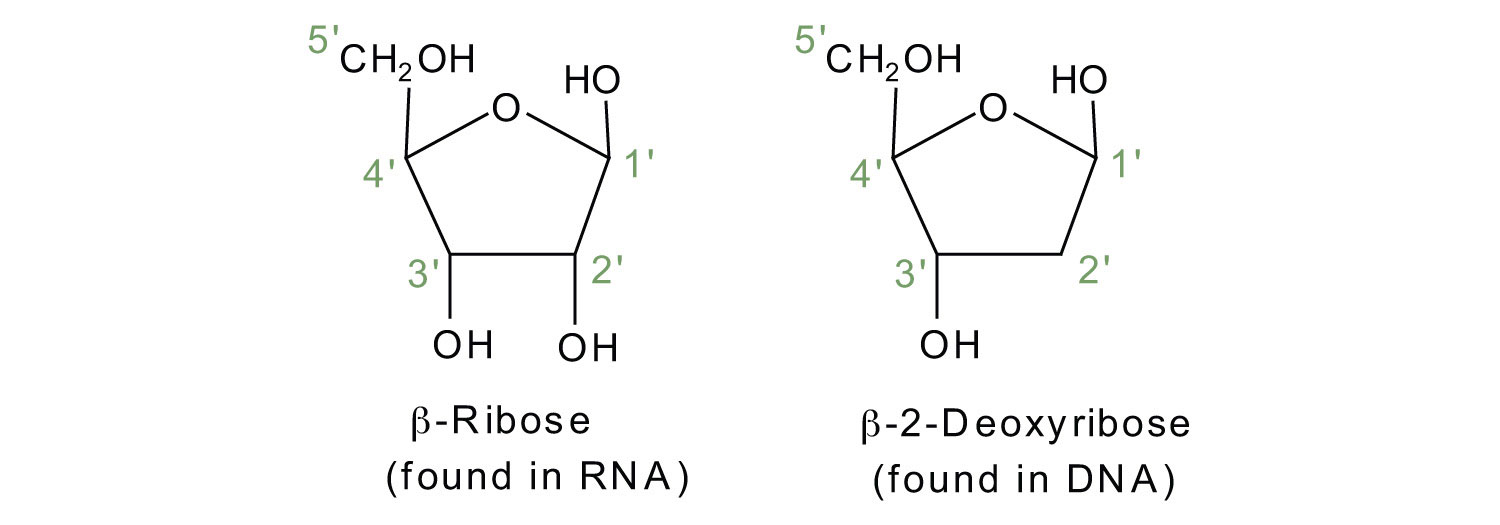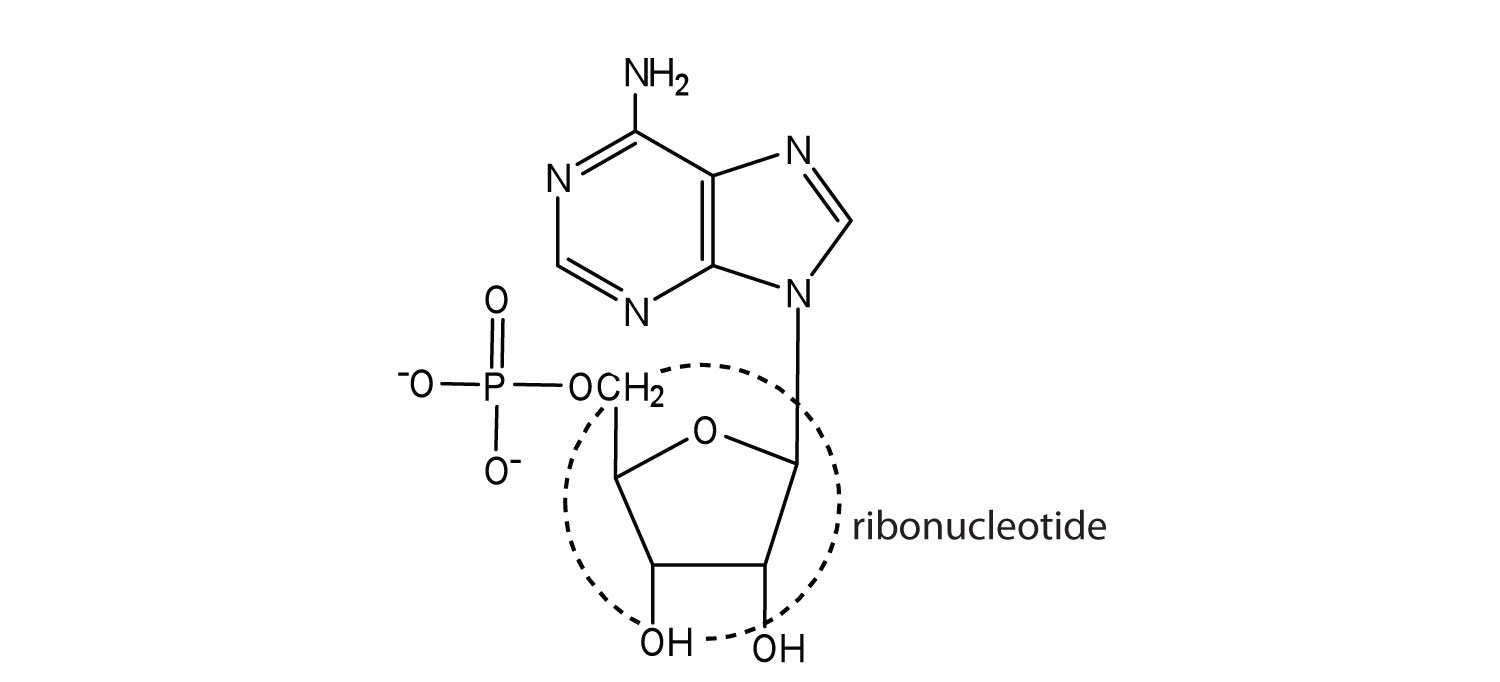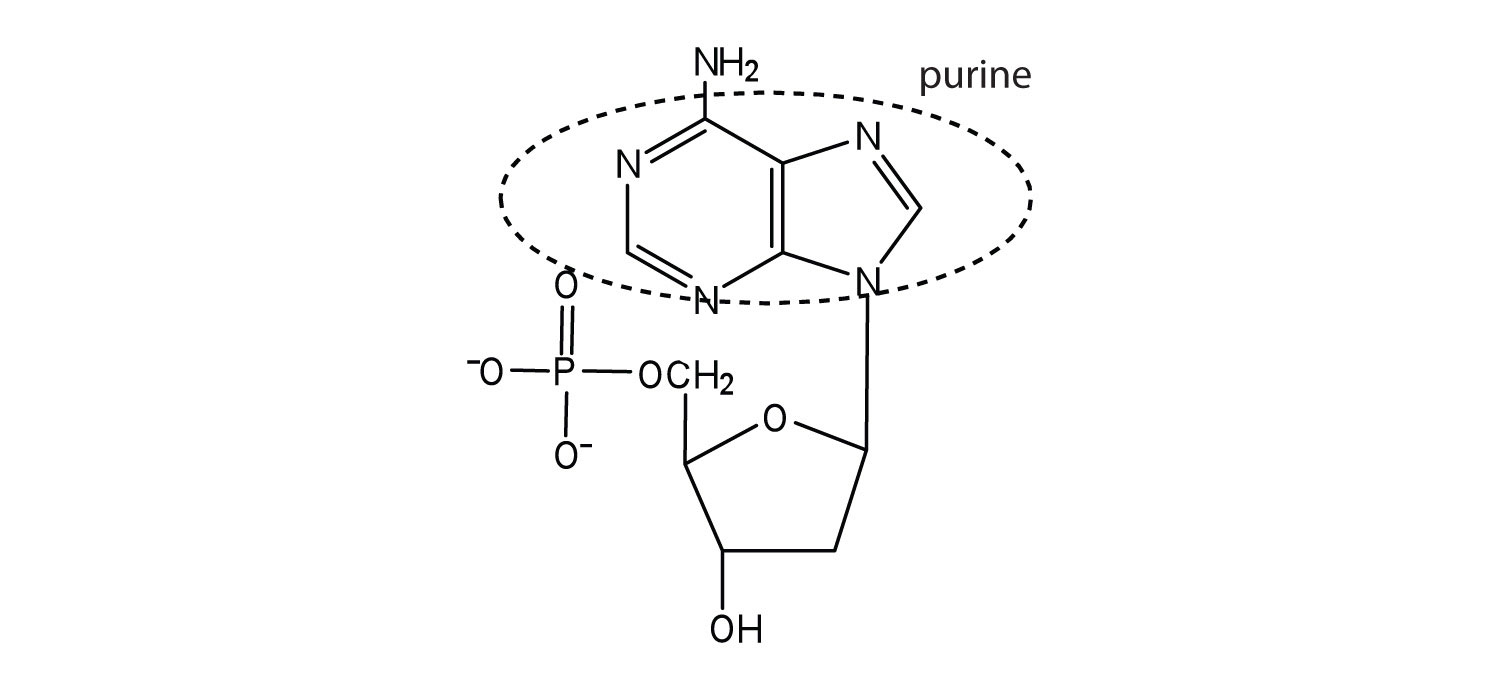14.4: Nucleotides and Nucleic Acids

Objectives

After completing this section, you should be able to

1. outline the relationship between nucleic acids, nucleotides and nucleosides.
2. identify, in general terms, the enzymatic hydrolysis products of nucleosides.
3. explain the structural difference between the sugar components of DNA and RNA.
4. identify by name the four heterocyclic amine bases found in deoxyribonucleotides.
5. identify by name the four heterocyclic amine bases found in ribonucleotides.
6. draw the general structure of a nucleotide and a nucleoside.
7. indicate the nitrogen atom by which a given purine or pyrimidine base attaches to the sugar component in nucleotides and nucleosides.
8. sketch a section of nucleic acid to show how the nucleotide units are joined together.

Key Terms

Make certain that you can define, and use in context, the key terms below.

• deoxyribonucleic acid (DNA)
• nucleosides nucleotides
• ribonucleic acid (RNA)

Study Notes

The five bases that are found in nucleotides are often represented by their initial letter: adenine, A; guanine, G; cytosine, C; thymine, T; and uracil, U. Note that A, G, C and T occur in DNA; A, G, C and U occur in RNA. You are not required to memorize the structures of these bases, but you must know how each one bonds to the sugar unit in a nucleotide.

To fulfill Objective 6, you should be able to reproduce the figure below.To fulfill Objective 7, you should study (but not necessarily memorize) Figure $$\PageIndex{2}$$ The Pyrimidine and Purine Nucleotides below.

The Learning Objective of this Module is to identify the different molecules that combine to form nucleotides.

The repeating, or monomer, units that are linked together to form nucleic acids are known as nucleotides. The deoxyribonucleic acid (DNA) of a typical mammalian cell contains about 3 × 109 nucleotides. Nucleotides can be further broken down to phosphoric acid (H3PO4), a pentose sugar (a sugar with five carbon atoms), and a nitrogenous base (a base containing nitrogen atoms).

$\mathrm{nucleic\: acids \underset{down\: into}{\xrightarrow{can\: be\: broken}} nucleotides \underset{down\: into}{\xrightarrow{can\: be\: broken}} H_3PO_4 + nitrogen\: base + pentose\: sugar} \tag{28.1.1}$

If the pentose sugar is ribose, the nucleotide is more specifically referred to as a ribonucleotide, and the resulting nucleic acid is ribonucleic acid (RNA). If the sugar is 2-deoxyribose, the nucleotide is a deoxyribonucleotide, and the nucleic acid is DNA.The nitrogenous bases found in nucleotides are classified as pyrimidines or purines. Pyrimidines are heterocyclic amines with two nitrogen atoms in a six-member ring and include uracil, thymine, and cytosine. Purines are heterocyclic amines consisting of a pyrimidine ring fused to a five-member ring with two nitrogen atoms. Adenine and guanine are the major purines found in nucleic acids (Figure $$\PageIndex{1}$$).Figure $$\PageIndex{1}$$: The Nitrogenous Bases Found in DNA and RNA

The formation of a bond between C1′ of the pentose sugar and N1 of the pyrimidine base or N9 of the purine base joins the pentose sugar to the nitrogenous base. In the formation of this bond, a molecule of water is removed. Table 28.1.1 summarizes the similarities and differences in the composition of nucleotides in DNA and RNA.

Example $$\PageIndex{1}$$

The numbering convention is that primed numbers designate the atoms of the pentose ring, and unprimed numbers designate the atoms of the purine or pyrimidine ring.

Table $$\PageIndex{1}$$: Composition of Nucleotides in DNA and RNA
Composition DNA RNA
pyrimidine bases cytosine and thymine cytosine and uracil
pentose sugar 2-deoxyribose ribose
inorganic acid phosphoric acid (H3PO4) H3PO4

The names and structures of the major ribonucleotides and one of the deoxyribonucleotides are given in Figure $$\PageIndex{2}$$.Figure $$\PageIndex{2}$$ The Pyrimidine and Purine Nucleotides

Apart from being the monomer units of DNA and RNA, the nucleotides and some of their derivatives have other functions as well. Adenosine diphosphate (ADP) and adenosine triphosphate (ATP), shown in Figure $$\PageIndex{3}$$, have a role in cell metabolism. Moreover, a number of coenzymes, including flavin adenine dinucleotide (FAD), nicotinamide adenine dinucleotide (NAD+), and coenzyme A, contain adenine nucleotides as structural components.Figure $$\PageIndex{3}$$: Structures of Two Important Adenine-Containing Nucleotides

Primary Structure of Nucleic Acids

Nucleotides are joined together through the phosphate group of one nucleotide connecting in an ester linkage to the OH group on the third carbon atom of the sugar unit of a second nucleotide. This unit joins to a third nucleotide, and the process is repeated to produce a long nucleic acid chain (Figure 28.1.4). The backbone of the chain consists of alternating phosphate and sugar units (2-deoxyribose in DNA and ribose in RNA). The purine and pyrimidine bases branch off this backbone.Figure $$\PageIndex{4}$$: Structure of a Segment of DNA. A similar segment of RNA would have OH groups on each C2′, and uracil would replace thymine.

Like proteins, nucleic acids have a primary structure that is defined as the sequence of their nucleotides. Unlike proteins, which have 20 different kinds of amino acids, there are only 4 different kinds of nucleotides in nucleic acids. For amino acid sequences in proteins, the convention is to write the amino acids in order starting with the N-terminal amino acid. In writing nucleotide sequences for nucleic acids, the convention is to write the nucleotides (usually using the one-letter abbreviations for the bases, shown in Figure 28.1.4) starting with the nucleotide having a free phosphate group, which is known as the 5′ end, and indicate the nucleotides in order. For DNA, a lowercase d is often written in front of the sequence to indicate that the monomers are deoxyribonucleotides. The final nucleotide has a free OH group on the 3′ carbon atom and is called the 3′ end. The sequence of nucleotides in the DNA segment shown in Figure 28.1.4 would be written 5′-dG-dT-dA-dC-3′, which is often further abbreviated to dGTAC or just GTAC.

Concept Review Exercises

1. Identify the three molecules needed to form the nucleotides in each nucleic acid.

1. DNA
2. RNA
2. Classify each compound as a pentose sugar, a purine, or a pyrimidine.

2. guanine
3. deoxyribose
4. thymine
5. ribose
6. cytosine

1. nitrogenous base (adenine, guanine, cytosine, and thymine), 2-deoxyribose, and H3PO4
2. nitrogenous base (adenine, guanine, cytosine, and uracil), ribose, and H3PO4
1. purine
2. purine
3. pentose sugar
4. pyrimidine
5. pentose sugar
6. pyrimidine

Key Takeaways

• Nucleotides are composed of phosphoric acid, a pentose sugar (ribose or deoxyribose), and a nitrogen-containing base (adenine, cytosine, guanine, thymine, or uracil).
• Ribonucleotides contain ribose, while deoxyribonucleotides contain deoxyribose.

Exercises

1. What is the sugar unit in each nucleic acid?

1. RNA
2. DNA
2. Identify the major nitrogenous bases in each nucleic acid.

1. DNA
2. RNA
3. For each structure, circle the sugar unit and identify the nucleotide as a ribonucleotide or a deoxyribonucleotide.

1.2.4. For each structure, circle the sugar unit and identify the nucleotide as a ribonucleotide or a deoxyribonucleotide.

1.2.5. For each structure, circle the nitrogenous base and identify it as a purine or pyrimidine.

1.2.6. For each structure, circle the nitrogenous base and identify it as a purine or pyrimidine.

1.2.1.2.1.2.